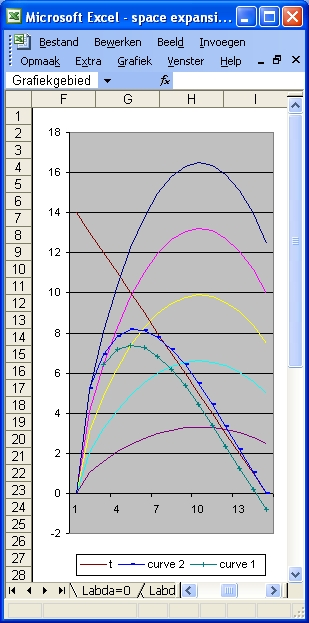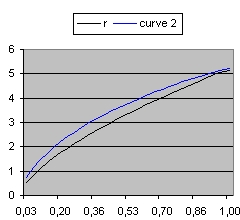### Answer question 2 - Labda = -0.04c = 60 Labda = -0.04 v0 = 3
Figure 1Figure 2 Detail Figure 2 shows the detail of Figure 1 in the range of 0 to 1 billion years. The black line represents the 100% distance line. The blue line shows the light ray starting from the Big Bang which reaches the Observer now. Figure 1 shows the condition when Labda = -0.04. In order to study what happens when Labda = -0.07 select this link: Friedmann L=-0.07
Back to Friedmann's Equation Question 2